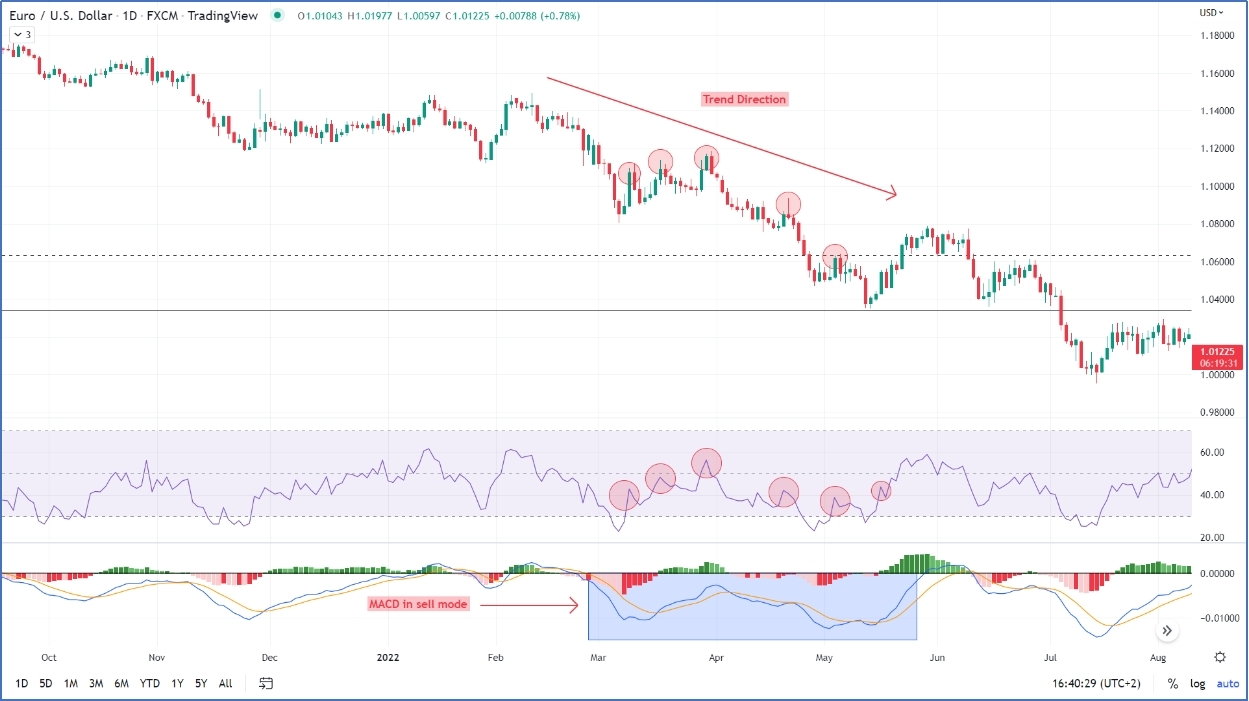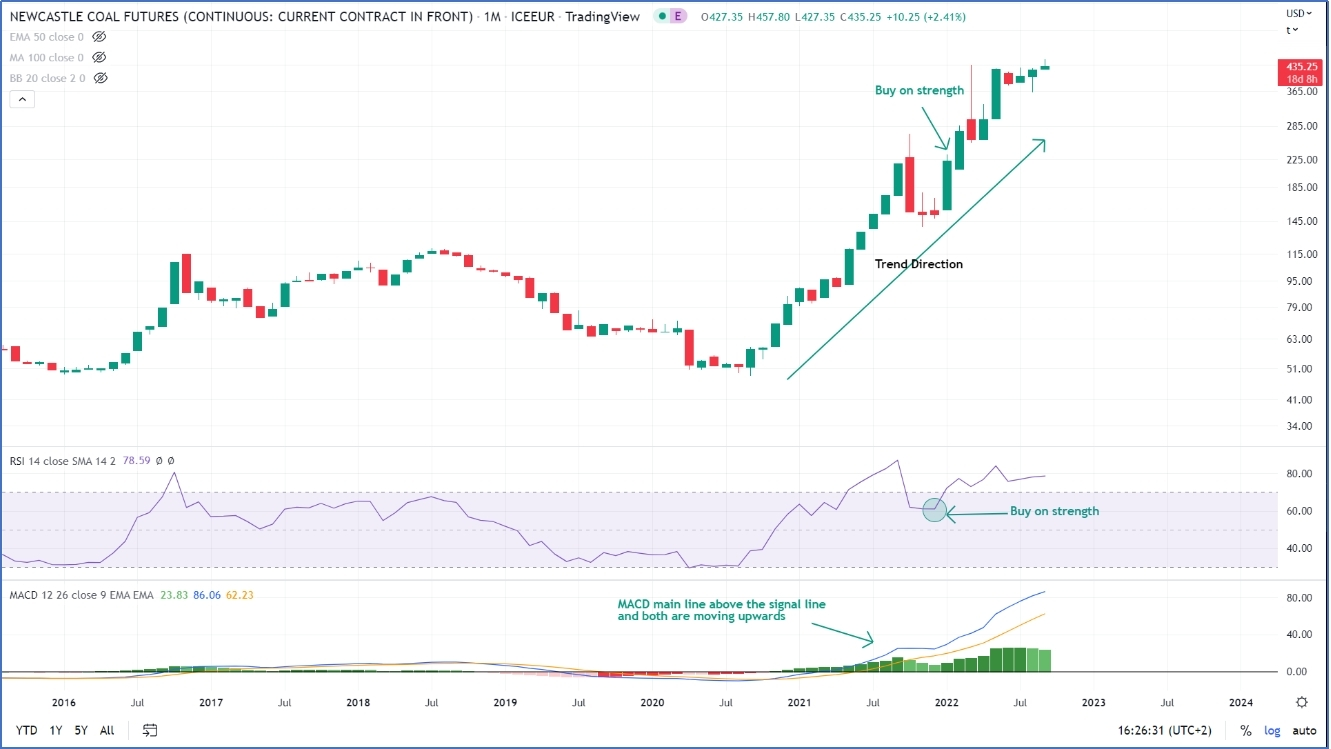# How To Combine Relative Strength Index With Moving Average Convergence Divergence

The MACD was invented by Gerald Appel. It is one of the well-known indicators that use moving averages.

### How to calculate the MACD:

The MACD consists of two lines, the first line is named the “main line” and it is constructed by subtracting the 26 exponential moving average from the 12-exponential moving average thus the main line of MACD= 12EMA – 26EMA. This means that if the 12EMA is greater than the 26 EMA the main line will be above the zero line and if the 12 EMA is smaller than the 26EMA the main line will be below the zero line.

The second line is called the “Signal line”, a 9 days exponential moving average for the main line I,e it is a moving average for a moving average.

Zero crossover: some technicians take buying decisions when the MACD line crosses above the zero line and sell when it crossed down the zero line, it is not recommended to rely only on the zero-line crossover as the entry might be too late. The zero crossovers can be used as a setup for trading using either buying or selling tactics.

Crossover between main line and signal line: taking a buy signal when the main line crosses over the signal line and sell when the main line crosses down the signal line. Traders might fall into the pitfall of false breaks, a crossover in the oversold or ould be better tactic to reduce false breaks.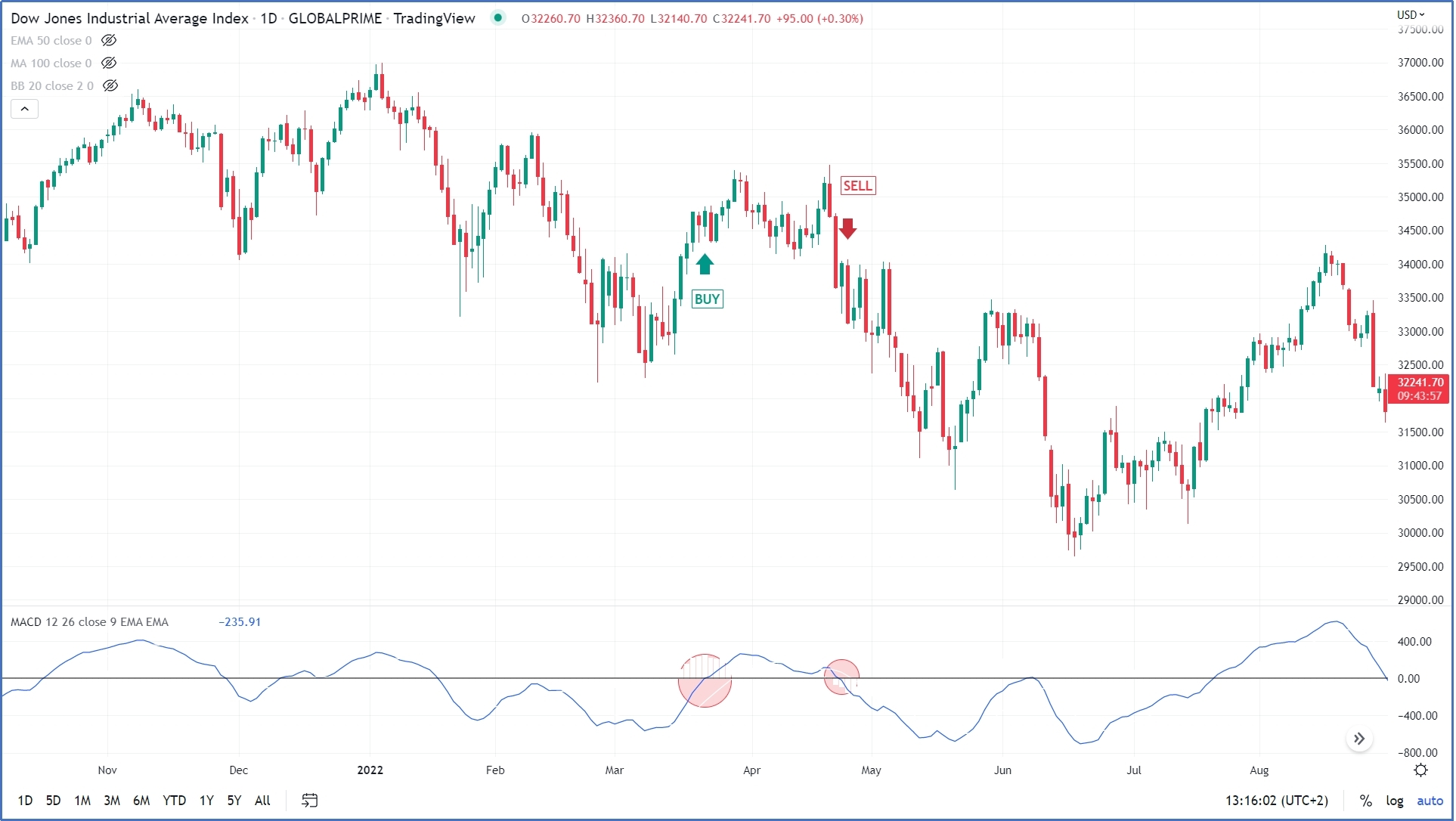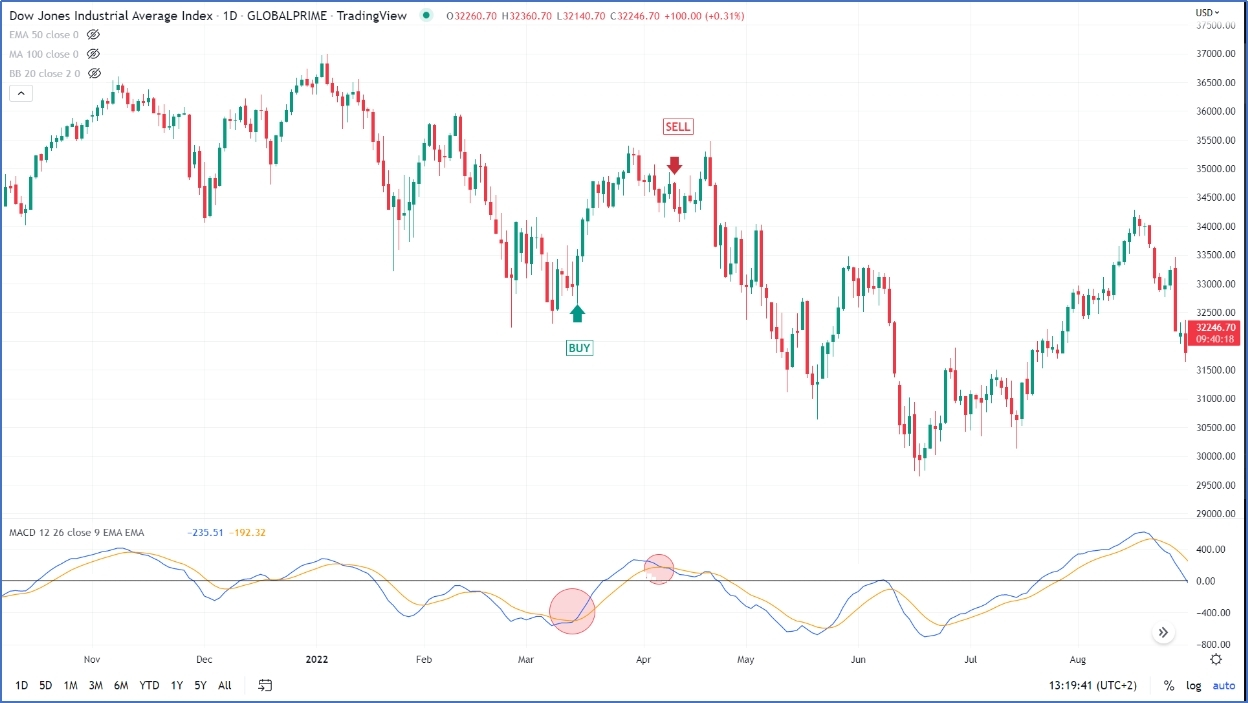### RSI indicator:

The relative strength index (RSI) is a technical momentum indicator that compares recent price gains against recent price losses, it is a momentum indicator measuring the magnitude of price movements. The RSI is a bounded indicator from zero to 100, reading above 70 usually indicates an overbought condition while readings below 30 usually indicate that the asset is in an oversold condition, that’s how it is utilized by traders. However, overbought and oversold assets do not necessarily rebound right away meaning that it is highly recommended to get confirmation from another indicator to verify the buy/sell decision and not to rely solely on RSI signals.

The RSI calculation formula: the RSI calculates the ratio of up closes to down closes over a specific amount of time. The resulting curve is an oscillator moving between 0 and 100. The formula of the RSI is as follows:

RSI= 100-(100/1+RS)

Where the RS is the average up closes of the last N days divided by the average down close of the same period.

1- Overbought/ oversold: the overbought is defined as when the indicator lies anywhere above the 70 levels and the oversold zone is when the indicator is anywhere below the 30 level. Accordingly, a simple strategy is to sell when the RSI crosses down the 70 levels and buy when the RSI crosses up the 30 levels.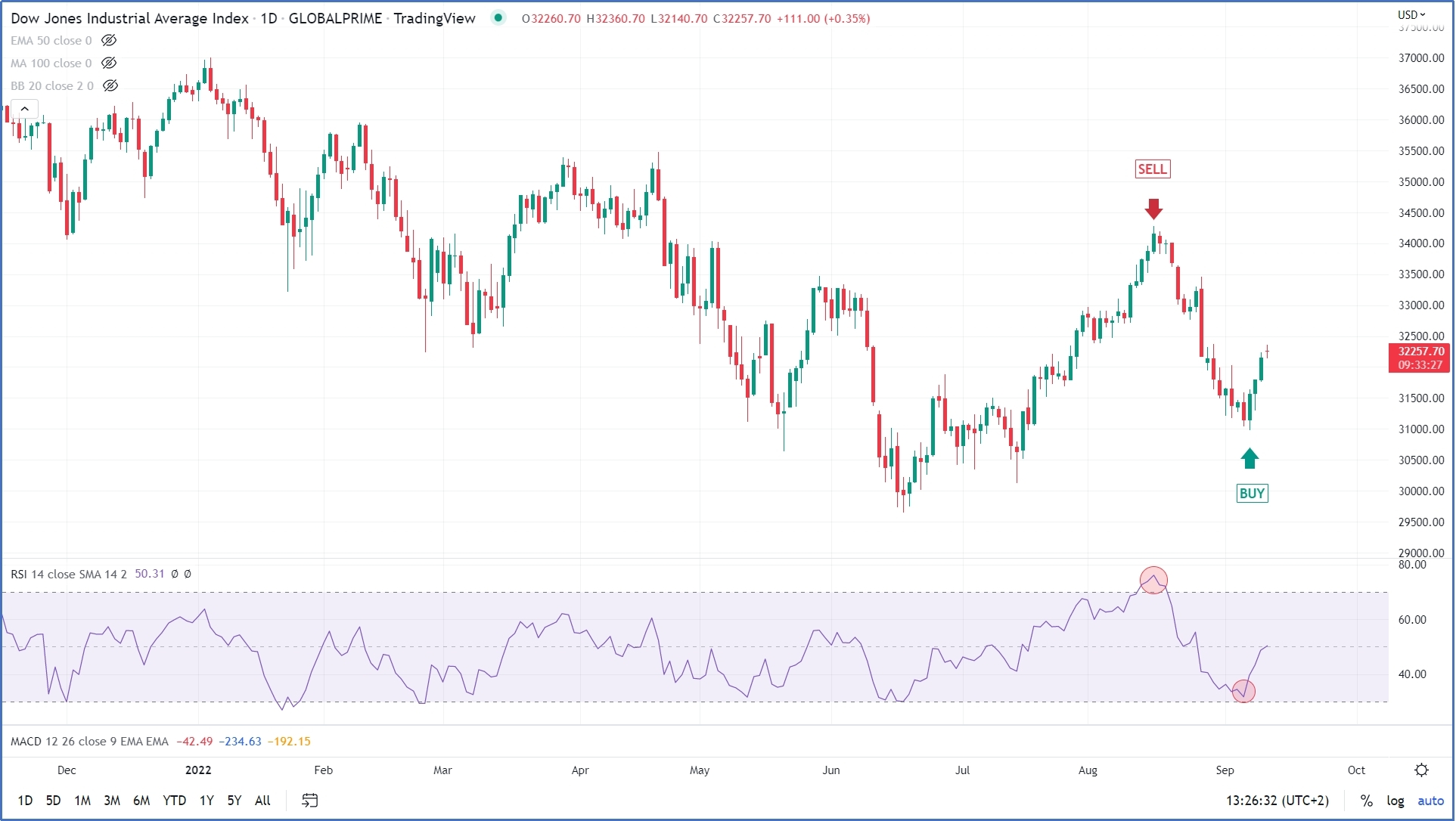2- Failure swing: a negative failure swing occurs when the RSI reaches the overbought area (over 70) declines and then rises again to a level below the first peak. The sell signal is triggered when the bottom connecting the two peaks is violated. A positive failure swing occurs when the RSI breaks the 30 to the downside, rises, and then declines to a level above the first bottom. An upside breaks of the peak connecting the two bottoms is a buy signal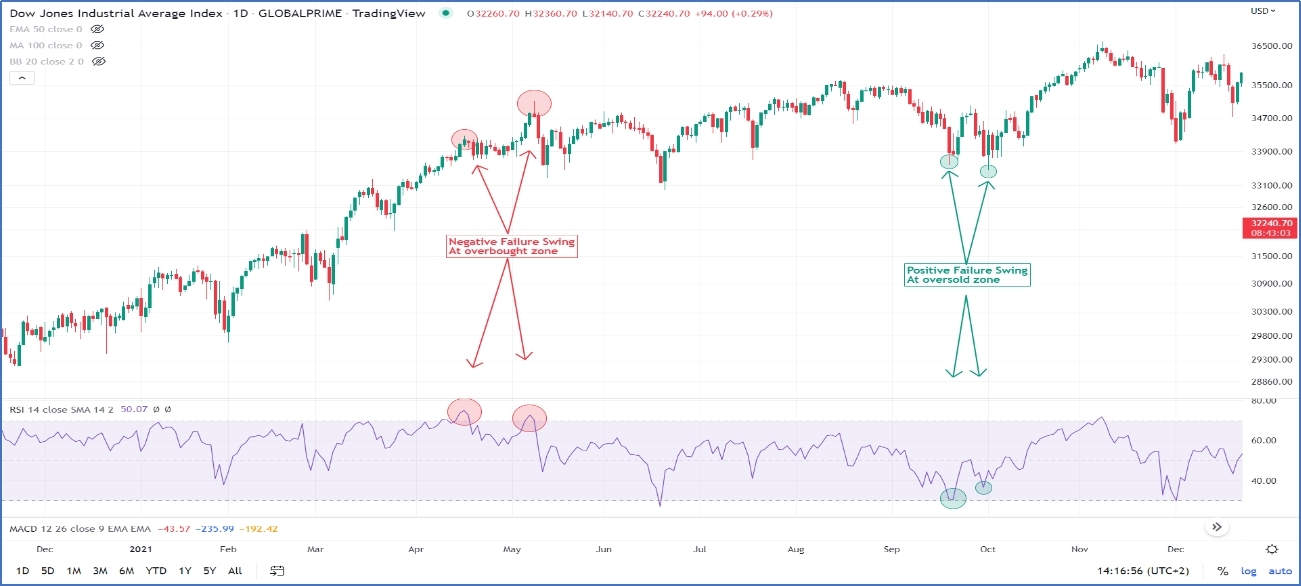3- Divergence: a positive diverge occurs when eh prices make a lower low, while RSI makes a higher low. Usually, divergences are stronger if occurred at oversold levels. A negative divergence occurs when prices make a higher high while the RSI makes lower high, negative divergences are stronger at overbought levels.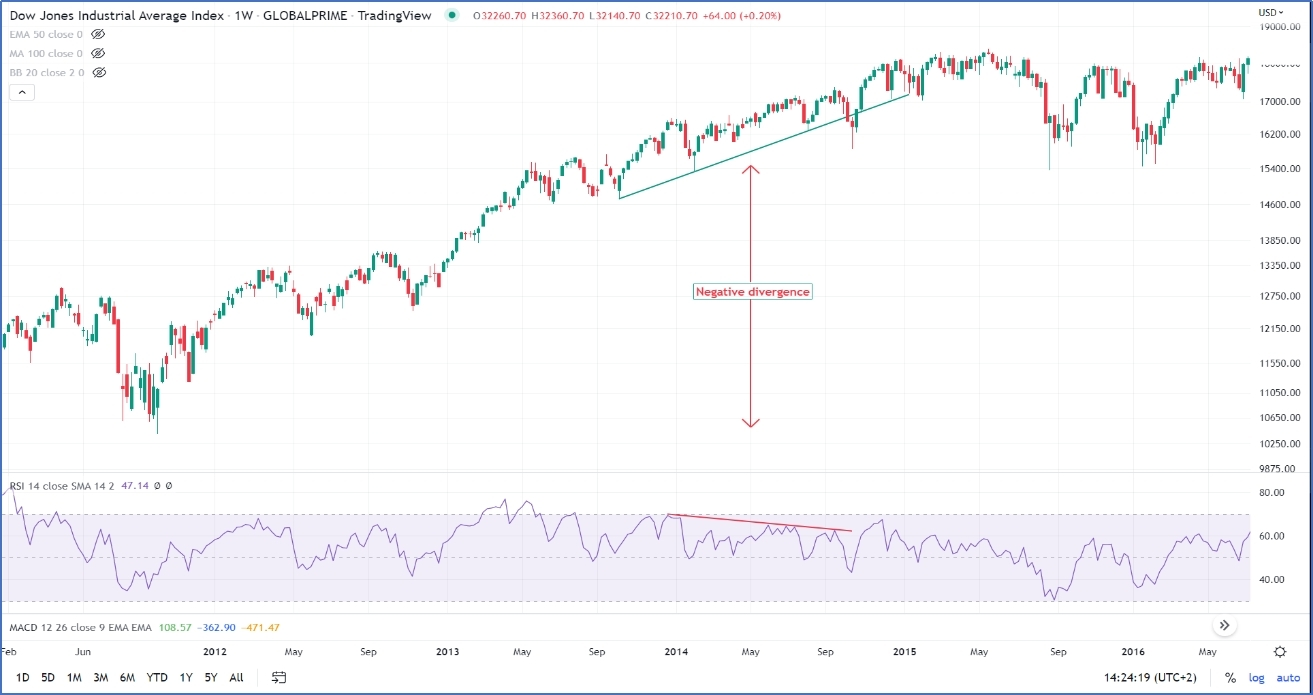### Combing MACD with RSI:

After demonstrating how to trade on each MACD and RSI separately, the following trading tactic highlights on how to combine both the MACD and RSI to identify better entry and exit points.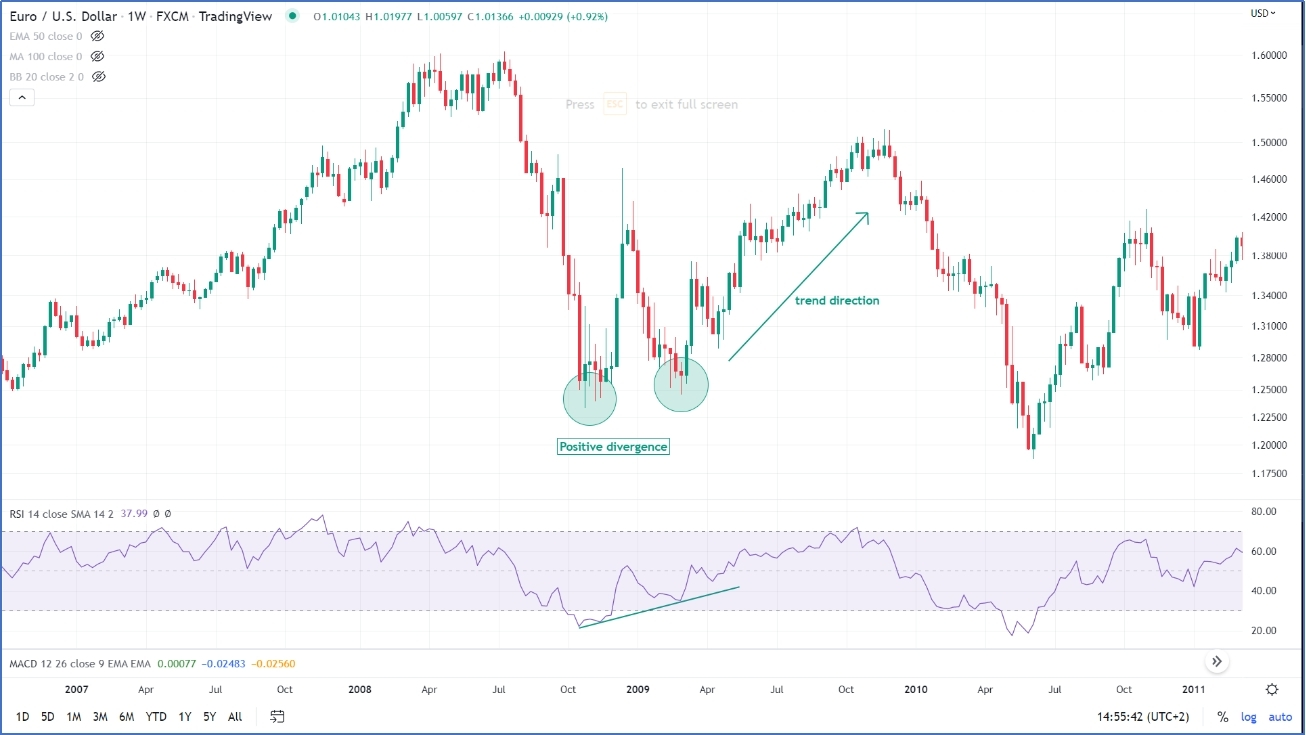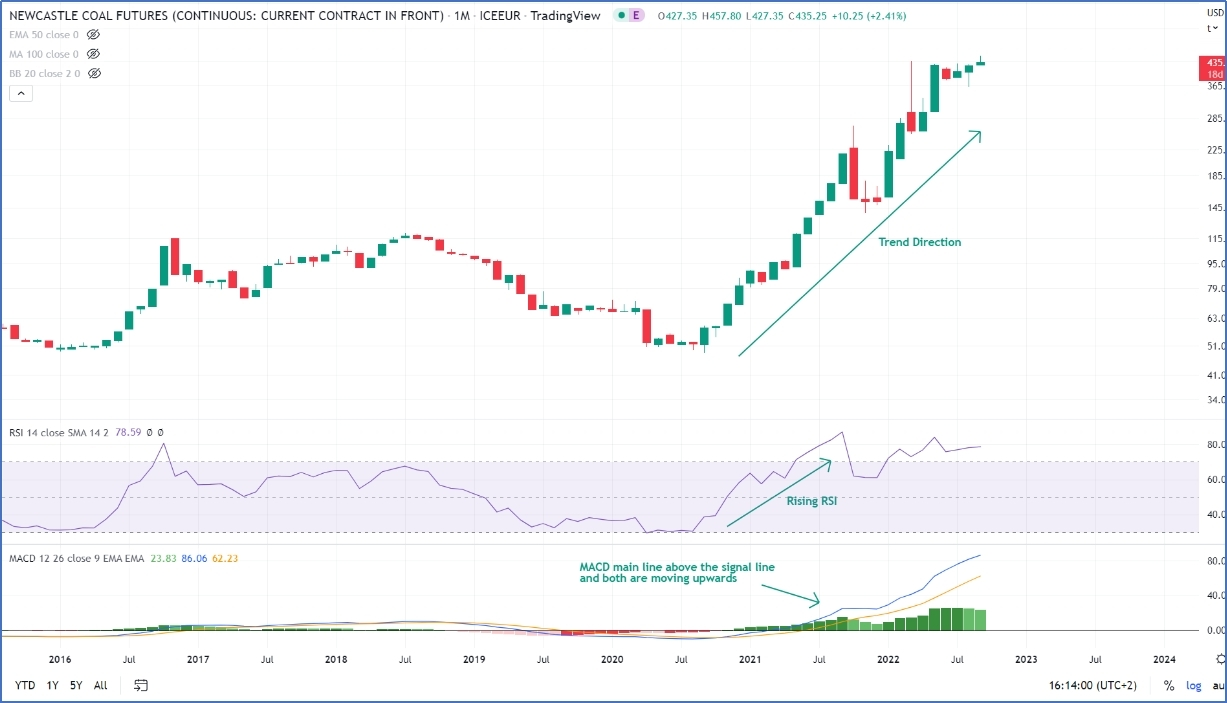The best RSI signal occurs when both MACD lines are rising and in a buy mode, meaning that the main line is above the signal line and both lines are rising. A rising MACD means the overall direction is up. A rising RSI indicates that a new upward move is expected in the direction of the trend, defined by the MACD. When the RSI buy signal occurs when the MACD is in a sell mode, it means that the next upward move is contra trend, thus increasing the risk of the trade.

Buy on strength: Buy on strength means that when the RSI is in an overbought zone while the MACD is in a buy mode (the main line is above the signal line and both lines are heading upward) then the RSI heads down below the overbought high/ peak and rebounds back, that is the [point of entry.